Home » GIS Analysis » How to Build Spatial Regression Models in ArcGIS

# How to Build Spatial Regression Models in ArcGIS### GIS Detectives Love Using Spatial Regression Models

The tech-savvy GIS detective loves spatial regression because it’s used to model spatial relationships. Regression models investigate what variables explain their location.

For example: If you have crime locations in a city, you can use spatial regression to understand the factors behind patterns of crime. We can use spatial regression to understand what variables (income, education, and more) explain crime locations.

A spatial regression model can then be used for decision making. For example, it can answer where are suitable locations for police stations. Spatial regression models are also used to predict future crime locations and even in other cities.

Let’s understand some of the terminologies in regression models.

• Dependent variable (Y): What are you trying to predict. (Location of crimes)
• Independent variable (X): Explanatory variables that explain the dependent variable. (Income, education, etc)
• Beta-coefficient: Weights reflecting the relationship between the explanatory and dependent variable.
• Residual: The value not explained by the model

Regression Formula:
y = β0 + (β1 × x1) + (β2 × x2) + … + (βn × xn) + Ε

### Spatial Regression Analysis in ArcGIS

Let’s put the ArcGIS regression tools in action by building a habitat suitability index (HSI) – also known as a resource selection function (RSF). With 308 GPS locations of marsh deer, we investigate the relationship between marsh deer and their landscape.

Important to note: This is a hypothetical scenario with made-up data.

• Which resources do marsh deer select or avoid?
• What are some of the factors that contribute to the location of marsh deer?
Habitat Suitability Index (HSI): An HSI is a numerical index that represents the capacity of a given habitat to support a selected species

Why create a HSI?
A land resource manager uses HSI to make better decisions on the landscape. If an HSI shows marsh deer prefer wetland habitat types, a land resource manager can preserve these types of habitat. A land resource manager can prohibit the development of infrastructure because an HSI shows the capacity of a given habitat to support marsh deer. HSI can be extrapolated to predict marsh deer in other locations.

### Explanatory Variables

What are the explanatory variables for marsh deer? This may be the most difficult part of regression modeling. We need to investigate potential habitat types for Marsh deer. This is where expert advice comes in handy. Here’s what we found:Marsh deer are found in marshy habitats such as floodplains, grasslands, and moist forests, preferring areas with a good amount of cover for protection, such as reed beds or where grass stands are high. This species is predominantly found close to permanent sources of water.WWF

Based on literature, Marsh deer select natural vegetation and water. But are there any land features that potentially disturb Marsh deer? We explore these independent variables using our spatial regression analysis.

### Independent and Dependent Variables

Our study area is characterized by natural vegetation and open water. A road cuts through cells A6-F6 which may act as a potential disturbance. Campgrounds are also present in cells B3, C7, and D7.

#### Marsh Deer Distribution and Campgrounds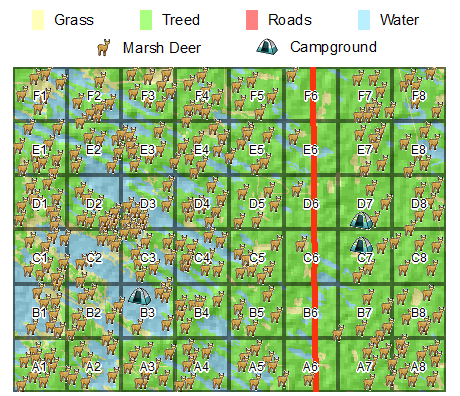Each dot represents the GPS position of marsh deer. Visually, there appears to be less marsh deer near roads and campgrounds. Another observation is that marsh deer appear denser in cells D2 and D3 where wetlands are present.

#### Hotspot Analysis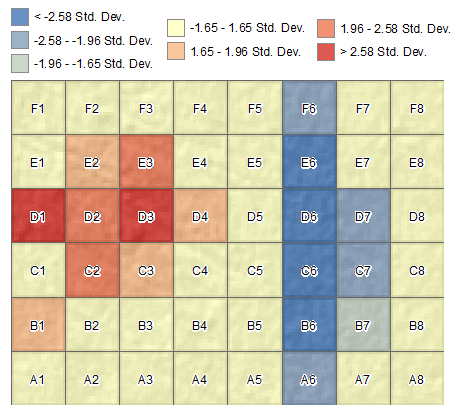This hotspot map confirms less deer close to roads to a degree of less than -2 standard deviations from the mean. Marsh deer are denser near cells D2. Other than these two low and hotspots, there doesn’t appear to be any more spatial patterns in the study area.

• Why are there so many deer in these hot spots?
• What are some of the factors that contribute to these hot spots?

These are the types of questions that can be answered using regression analysis. Let’s use spatial regression to model spatial relationships between marsh deer and land features.

### Ordinary Least Square (OLS) Regression

The first step is to group the independent and dependent variables per grid cell. We cannot look at the Marsh deer locations as points. The table must have the number of deers, campgrounds, and wetlands for each grid cell. The table below is an example of a pre-processed table using OLS.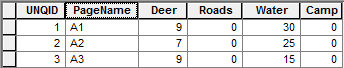We will use the “Ordinary Least Squares Regression” tool in the “Modelling Spatial Relationships” toolkit.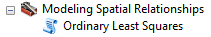Ordinary Least Square Regression Model:
Input Feature Class: Grid cells with aggregated data
Unique ID: A unique ID field (ex, 1, 2, 3…)
Output Feature Class: Path and name of output
Dependent Variable: Deer count
Explanatory Variables: Campgrounds, roads and Water
Output report file: Generates a report file.

After running the OLS tool, the residuals of the prediction model will be added to your display. The residuals are essentially the error in the model.

Let’s take a closer look at what a residual actually is before moving forward. If we look at cell A1 (bottom left), there were 9 deer found in this grid cell. The OLS model built weights based on the amount of trees, wetlands, grass, roads, and campgrounds in the cell. These weights are the beta-coefficient values. When the weights were plugged into the regression formula, there was an estimated 6.98 deer in cell A1. When you subtract 6.98 from 9, we get a residual of 2.01. In other words, the model underpredicts the actual value by 2.01.

#### Ordinary Least Squares Regression Residuals Values: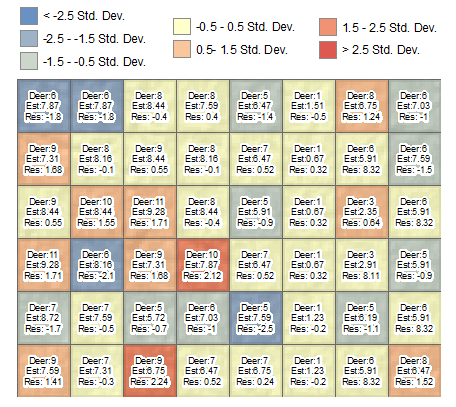Variable Beta-coefficient p VIF Intercept β0 = 5.916744 0.0000001* —– Roads β1 = -0.524393 0.0000001* 1.150233 Water β2 = 0.056088 0.0000001* 1.139367 Camp β3 = -3.558805 0.0000001* 1.010354

The low negative beta-coefficient of campgrounds (-3.56) can be interpreted as areas where marsh deer avoid. Roads as well had a negative value of -0.52, meaning deer do not select these grids. Marsh deer prefer wetlands as a suitable habitat. This model confirms this belief.

We can manually plug in the beta-coefficient model into the regression model. The result is the predicted value. In our case, it is the predicted number of deer in the grid cell.

y = β0 + (β1 × x1) + (β2 × x2) + … + (βn × xn) + Ε

A1 = 5.916744 + (-0.524393 × 0) + (0.056088 × 30) + (-3.558805 × 0)

A1 = 7.59

This OLS model achieves an adjusted R-squared value of 0.795. With these 3 factors, we can explain 79.5% of the variation that’s occurring.

What is the model missing? Known predators, forest age, wetland type – include other facets of

#### Variance Inflation Factor (VIF):

Another statistic of interest is the Variance Inflation Factor (VIF). If the VIF > 7.5, this indicates redundancy among explanatory variables. Our HSI model satisfied these criteria with VIF

#### Probability and Robust Probability:

An asterisk (*) indicates that the coefficient is statistically significant (p

#### Jarque-Bera Statistic:

When this test is statistically significant (p

### Moran’s I Spatial Autocorrelation

The spatial autocorrelation will tell us if the under/over predictions are random. No model can predict perfectly and will always over and underpredict. Spatial autocorrelation investigates if the OLS model is randomly distributed.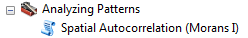Moran’s I Spatial Autocorrelation:
Input Feature Class: OLS output
Input Field: Standard Residual (StdResid)
Generate Report: YES

When you click OK, a report will be generated. Double-click the report, and ensure that the results are random.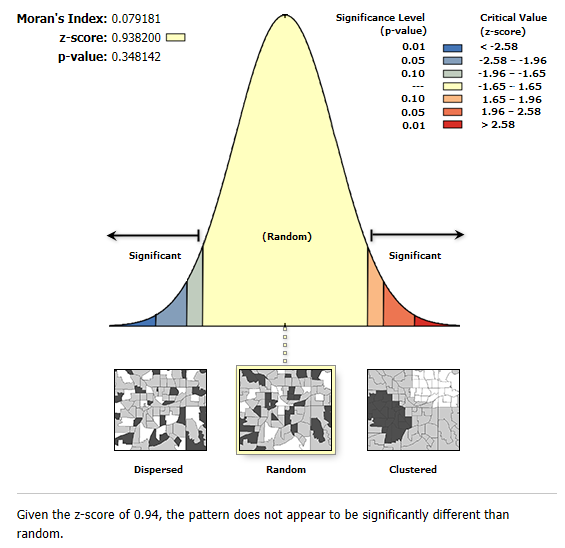### Case Closed?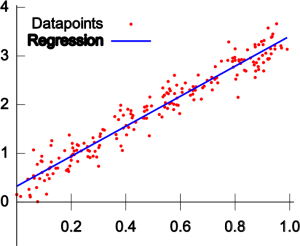The ArcGIS spatial regression tool was used to build a spatial relationship between Marsh deer, campgrounds, roads, and wetlands. Regression tools investigated the relationship between these factors and generate weights for each variable.

These weights were plugged into the regression formula to calculate and predict the number of deer. The variance inflation factor, z-scores, Jarque-Bera and Moran’s I ensured robustness and statistical significance in the spatial regression model.

The regression model shows how Marsh deer select wetlands as a suitable habitat. It also shows that Marsh deer tend to avoid campgrounds and roads.

This is useful to land resource managers to potentially restrict the development of campgrounds and roads to conserve this type of deer. The regression model can also be used to predict Marsh deer in other areas.